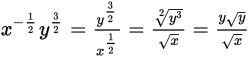# SAT Math Multiple Choice Question 887: Answer and Explanation

### Test Information

Question: 887

2. Which of the following gives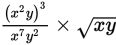written in simplest form?

• A.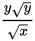• B.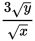• C.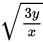• D.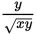Explanation:

A

Difficulty: Hard

Category: Passport to Advanced Math / Exponents

Strategic Advice: Before you can combine radical expressions with non-radical expressions, you must write them in the same form. Here, you can rewrite the square root of xy as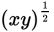.

Getting to the Answer: First, simplify as much as possible using the rules of exponents. Then rewrite the radical as a power of xy and simplify further, using the rules of exponents to combine terms that have the same base: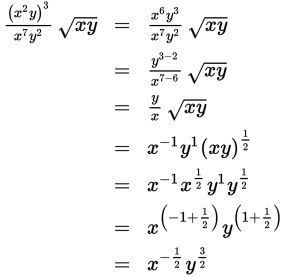The answer choices are given as radicals, so change the powers back to roots. The negative power on x moves it to the denominator, and for the y term, use the saying "power over root" to help you write the correct radical form: